Technology

# An Introduction to Computability Theory and Complexity

With experience at Google, Exxon, and a Master's in CS, Mehmet's deep expertise serves him well as a full-stack javascript developer.
Read the Spanish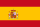version of this article translated by Marisela Ordaz

Have you ever wondered: What exactly is the device that you are reading this article on? What is a computer?

Computational science dates back to a time long before these modern computing devices were even imagined. In an industry where the more frequently asked questions revolve around programming languages, frameworks, and libraries, we often taken for granted the fundamental concepts that make a computer tick.

But these computers, which seem to possess endless potential—do they have any limitations? Are there problems that computers cannot be used to solve?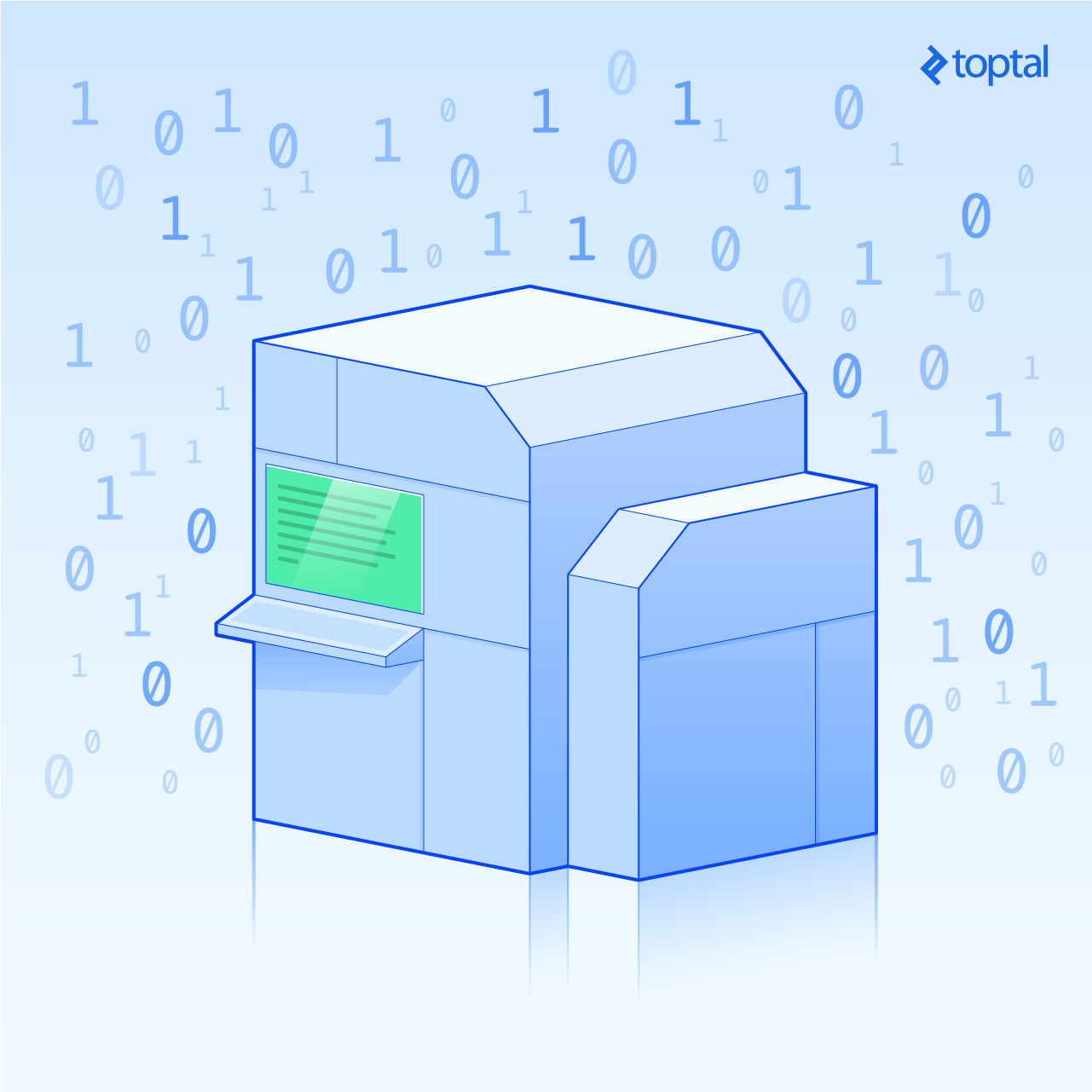In this article, we will address these questions by stepping away from the particulars of programming languages and computer architectures. By understanding the power and limitations of computers and algorithms, we can improve the way we think and better reason about different strategies.

The abstract view of computing produces results that have stood the test of time, being as valuable to us today as they were when initially developed in the 1970s.

## Computability

### What Is a Computer? What Is a Problem?

In school, we are often taught a mental model of problems and functions that goes something like this:

A function is a procedure you apply to an input x in order to find an output f(x).

Turns out the mathematical definition is different:

A function is a set of ordered pairs such that the first element of each pair is from a set X (called the domain), the second element of each pair is from a set Y (called the codomain or range), and each element of the domain is paired with exactly one element of the range.

That was quite the mouthful. But, what exactly does that mean?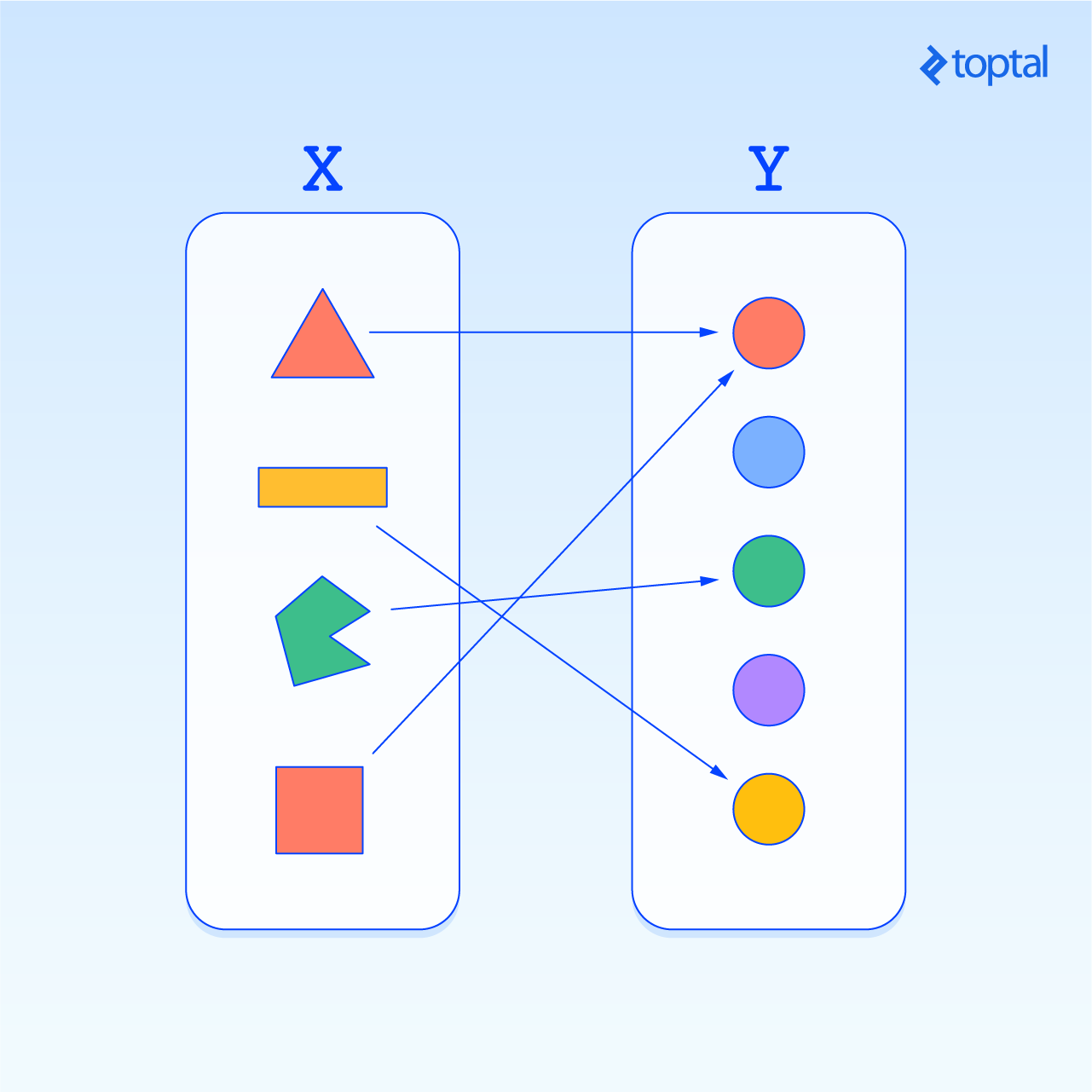This definition tells us that a computer is a machine for computing functions.

Why?

Because computers transform arbitrary input to some output. In other words, they solve problems. The two definitions of functions, the one we are so familiar with and the formal one, coincide for many practical purposes.

However, the mathematical definition allows us to reach interesting conclusions such as the existence of uncomputable functions (i.e., unsolvable problems):

Because, not every function can be described as an algorithm.

#### Rules of the Game

To help make our arguments, let us imagine computers as machines taking some input, performing a sequence of operations, and after some time, giving some output.

We will call the input the machine’s alphabet; that is, a set of strings of characters from some finite set. For example, the machine’s alphabet may be binary (0s and 1s) or it might be the ASCII character set. Any finite sequence of characters is a string—for example, “0110.”

Furthermore, we will represent a machine’s output as a binary accept-reject decision, delivered once the machine (hopefully) finishes its computation. This abstraction fits well with the mathematical definition of functions from earlier.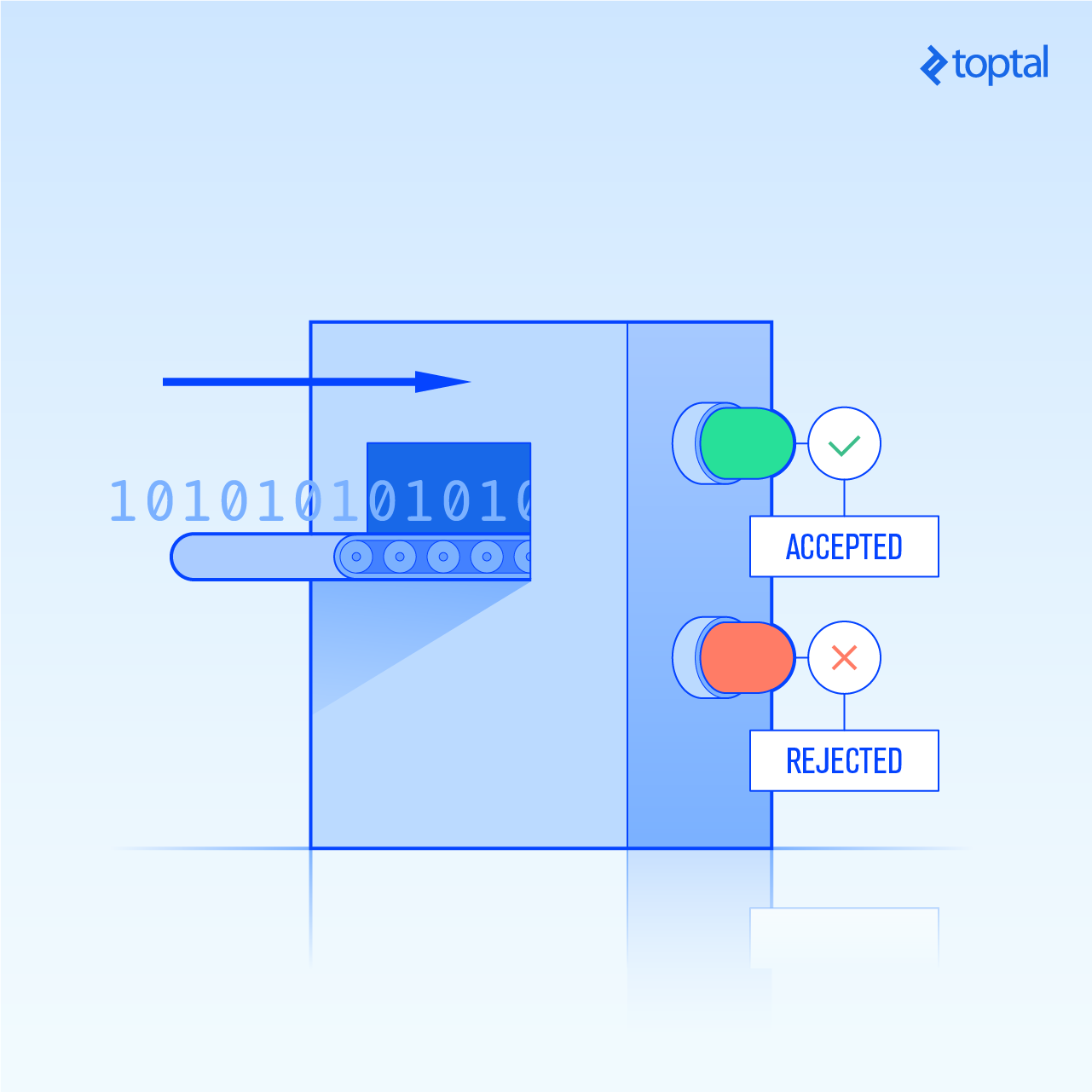Given these parameters, it is important to characterize one more type: a collection of strings. Maybe we care about the set of strings that some machine accepts, or maybe we are building a machine that accepts strings in a certain set and no others, or maybe we’re asking if it’s even possible to design a machine that accepts everything in some particular set and no others.

In all these cases, a set of strings is called a language—for example, the set of all binary strings that represent even numbers or the set of strings that have an even number of characters. It turns out that languages, like numbers, may be operated on with operators such as concatenation, union, intersection, and the like.

One important operator is the Kleene star operator that’s also used with regular expressions. This can be thought of as the union of all possible powers of the language. For example, if our language A is the set of strings { ‘01’, ‘1’ }, then one member of A* is the string ‘0101111’.

### Countability

The last piece of the puzzle before we prove our claim that not all functions are computable is the concept of countability. Intuitively, our proof will show that there are more languages; that is, more problems than there are possible programs to solve them. This works because the question of whether a string belongs in a language (Yes/No) is itself a problem.

More precisely, our proof claims that the set of possible programs is countably infinite while the set of languages over an alphabet is uncountably infinite.

At this point, you may be thinking, “Infinity is a strange enough idea by itself; now I have to deal with two of them!”

Well, it’s not that bad. A countably infinite set is one that can be enumerated. It’s possible to say this is the first element, this is the second element, and so forth, eventually assigning a number to every element of the set. Take the set of even numbers, for example. We can say that 2 is the first one, 4 the second, 6 the third, and so forth. Such sets are countably infinite or countable.

With some sets though, like the real numbers, it doesn’t matter how clever you are; there simply is no enumeration. These sets are uncountably infinite or uncountable.

#### Countably Many Programs

First, we want to show that the set of computer programs is countable. For our purposes, we do this by observing that the set of all strings over a finite alphabet is countable. This works because computer programs are finite strings themselves.

The proof is straightforward, and we don’t cover the details here. The key takeaway is that there are just as many computer programs out there as there are, say, natural numbers.

To reiterate:

The set of all strings over any alphabet (e.g., set of all computer programs) is countable.

#### Uncountably Many Languages

Given this conclusion, what about the subsets of these strings? Asked another way, what about the set of all languages? It turns out that this set is uncountable.

The set of all languages over any alphabet is uncountable.

Once again, we don’t cover the proof here.

#### Consequences

Although they may not be immediately apparent, the consequences of the uncountability of languages and the countability of the set of all computer programs are profound.

Why?

Suppose A is the set of ASCII characters; ASCII characters are just those needed to compose a computer program. We can see that the set of strings that represent, say, JavaScript programs, is a subset of A* (here, * is the Kleene star operator). The choice of JavaScript is arbitrary. Since this set of programs is a subset of a countable set, we have that the set of JavaScript programs is countable.

In addition, let us consider that for any language L, we can define some function f that evaluates to 1 if some string x is in L and 0 otherwise. All such functions are distinct. Because there is a 1:1 correspondence with the set of all languages and because the set of all languages is uncountable, we have that the set of all such functions is uncountable.

Here is the profound point:

Since the set of all valid programs is countable but the set of functions is not, then there must be some functions for which we simply cannot write programs.

We don’t yet know what these functions or problems look like, but we know they exist. This is a humbling realization, because there are some problems out there for which there is no solution. We consider computers to be extremely powerful and capable, yet some things are out of even their reach.

Now the question becomes, “What do these problems look like?” Before we continue describing such problems, we have to first model computation in a generalized way.

### Turing Machines

One of the very first mathematical models of a computer was developed by Alan Turing. This model, called the Turing machine, is an extremely simple device that completely captures our notion of computability.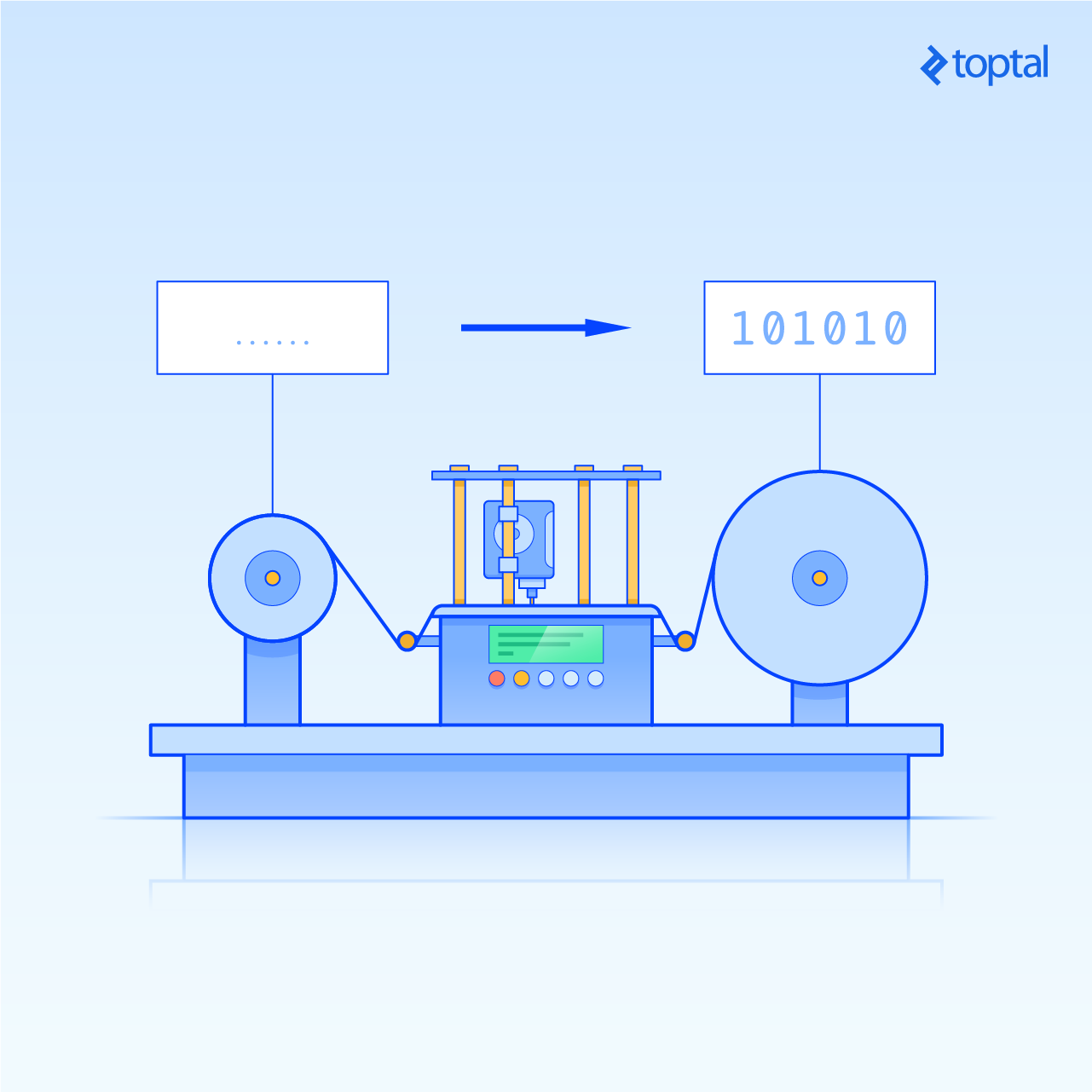The input to the machine is a tape onto which the input has been written. Using a read/write head, the machine turns input into output through a series of steps. At each step, a decision is made about whether and what to write to the tape and whether to move it right or left. This decision is based on exactly two things:

• The current symbol under the head, and

• The machine’s internal state, which is also updated as the symbol is written

That’s it.

#### Universality

In 1926, Alan Turing not only developed the Turing machine but also had a number of other major insights into the nature of computation when he wrote his famous paper, “On Computable Numbers.” He realized that a computer program itself could be considered input to a computer. With this point of view, he had the beautiful idea that a Turing machine could simulate or execute that input.

While we take these ideas for granted today, back in Turing’s day, the idea of such a universal machine was the major breakthrough that allowed Turing to develop unsolvable problems.

#### Church-Turing Thesis

Before we continue, let’s examine an important point: We know the Turing machine is a model of computation, but is it general enough? To answer this question, we turn to the Church-Turing Thesis, which gives credence to the following statement:

Everything computable is computable by a Turing machine.

While Turing developed the Turing machine as a model of computation, Alonzo Church also developed a model of computation known as lambda-calculus. These models are powerful, because they both describe computation and do so in a way equal to any of today’s computers or for that matter any computer ever. This means we can use a Turing machine to describe the unsolvable problems we seek, knowing that our findings would apply to all possible computers past, present, and beyond.

#### Recognizability & Decidability

We have to cover just a bit more background before we concretely describe an unsolvable problem, namely the concepts of language recognizers and language deciders.

A language is recognizable if there is a Turing machine that recognizes it.

and

A language is decidable if there is a Turing machine that decides it.

To be a recognizer of a language, a Turing machine must accept every string in the language and it must not accept anything not in the language. It may reject or loop on such strings. To be a decider, a Turing machine must always halt on its input either by accepting or by rejecting.

Here, the idea of halting on input is critical. In fact, we see that deciders are more powerful than recognizers. Furthermore, a problem is solvable, or put another way, a function is decidable only if there exists a Turing machine that decides the language described by the function.

#### Undecidability

If you’ve ever written a computer program, surely you must know the feeling of sitting there just watching the computer spin its wheels when the program is executed. You don’t know whether the program is just taking a long time or if there is some mistake in the code causing an infinite loop. You may have even wondered why the compiler doesn’t check the code to see if it would stop or loop forever when run.

The compiler doesn’t have such a check because it simply can’t be done. It’s not that compiler engineers aren’t smart enough or lack the resources; it is simply impossible to check an arbitrary computer program to determine whether it halts.

We can prove this using the Turing machine. Turing machines can be described as strings, so there are a countable number of them. Suppose M1, M2, and so on make up the set of all Turing machines. Let us define the following function:

f(i, j) = 1 if Mi accepts <Mj>, 0 otherwise

Here, <M> is the syntax for “string encoding of M,” and this function represents the problem of outputting 1 if Mi halts by accepting Mj as input and outputting 0 otherwise. Note that Mi must halt (i.e., be a decider). This is required since we wish to describe an undecidable function (i.e., unsolvable problem).

Now, let us also define a language L that consists of string encodings of Turing machines that do NOT accept their own descriptions:

L = { <M> | M does not accept <M> }

For example, some machine M1 may output 0 on the input <M1> while another machine M2 may output 1 on the input <M2>. To prove this language is undecidable, we ask what ML, the machine that decides the language L, does when it is given its own description <ML> as input. There are two possibilities:

ML accepts <ML>

or

ML rejects <ML>

If ML accepts its own encoding, then that means <ML> is not in the language L; however, if that were the case, then ML should not have accepted its encoding in the first place. On the other hand, if ML does not accept its own encoding, then <ML> is in the language L, so ML should have accepted its string encoding.

In both cases, we have a paradox, or in mathematical terms, a contradiction, proving that the language L is undecidable; thus, we’ve described our first unsolvable problem.

#### Halting Problem

While the problem we just described may not seem relevant, it can be reduced to additional unsolvable problems of practical importance, most notably the halting problem:

The language of encodings of Turing machines that halt on the empty string.

The halting problem applies to the question of why compilers cannot detect infinite loops from earlier. If we cannot determine whether a program terminates on the empty string, then how could we possibly determine if its execution would result in an infinite loop?

At this point, it might seem like we just waved our hands around to reach some simple conclusion; however, we actually realized that no Turing machine can tell whether a computer program will halt or remain in a loop forever. This is an important problem with practical applications, and it can’t be solved on a Turing machine or any other kind of computer. An iPhone cannot solve this problem. A desktop with many cores cannot solve this problem. The cloud cannot solve this problem. Even if someone were to invent a quantum computer, it still wouldn’t be able to solve the halting problem.

### Summary

In our examination of computability theory, we have seen how there are many functions that are not computable in any ordinary sense of the word by a counting argument. We precisely defined what we mean by computation, going all the way back to Turing’s inspiration from his own experience with pen and paper to formalize the Turing machine. We have seen how this model can compute anything that any computer today or envisioned for tomorrow can, and we realized a class of problems that are not computable at all.

Still, computability has a downside. Just because we can solve a problem doesn’t mean we can solve it quickly. After all, what good is a computer if its computation isn’t going to finish before the sun goes nova on us tens of millions of years in the future?

Leaving computable functions and languages behind, we now discuss computation complexity, surveying efficient computation and the famous P vs. NP problem.

## Complexity

### Slow vs. Fast

Computer scientists recognize a variety of classes of problems, and two classes that we care about include problems that computers can solve quickly or efficiently known as P and problems whose solutions can be verified quickly but cannot be obtained quickly known as NP.

For example, suppose you are responsible for developing algorithms for an online dating service and someone poses the question, “Can everyone get a date?” The answer boils down to pairing compatible individuals such that everyone is paired. Turns out there are efficient algorithms for solving this problem. This problem is in the set P.

Well, what if we wanted to identify the largest clique among our users? By clique, we mean the largest network of individuals that are all compatible with one another. When the user count is low, this problem can be solved quickly. We can easily identify, say, a clique with 3 users. As we start to look for larger cliques, however, the problem becomes harder and harder to solve. This problem is in the set NP.

### Formal Definitions

P is the set of problems that are solvable in polynomial time. That is, the number of computational steps is bounded by a polynomial function with respect to the problem size. We know that the “Can everyone get a date?” question, also known as bipartite matching problem, is in P.

NP is the set of problems that are verifiable in polynomial time. This includes every problem in P, of course; however, we don’t know whether this containment is strict. We know of problems that are efficiently verifiable but not efficiently solvable, but we don’t know if the problem is truly intractable. The clique problem is one such problem. We know we can verify the solution efficiently, but we don’t know for sure if we can solve the problem efficiently.

Lastly, NP-complete is the set of problems that are the hardest problems in NP. They are referred to as the hardest because any problem in NP can efficiently be transformed into NPC. As a result, if someone were to identify an efficient solution to a problem in NPC, then the entire class of NP would be absorbed by P. The clique problem is also in NPC.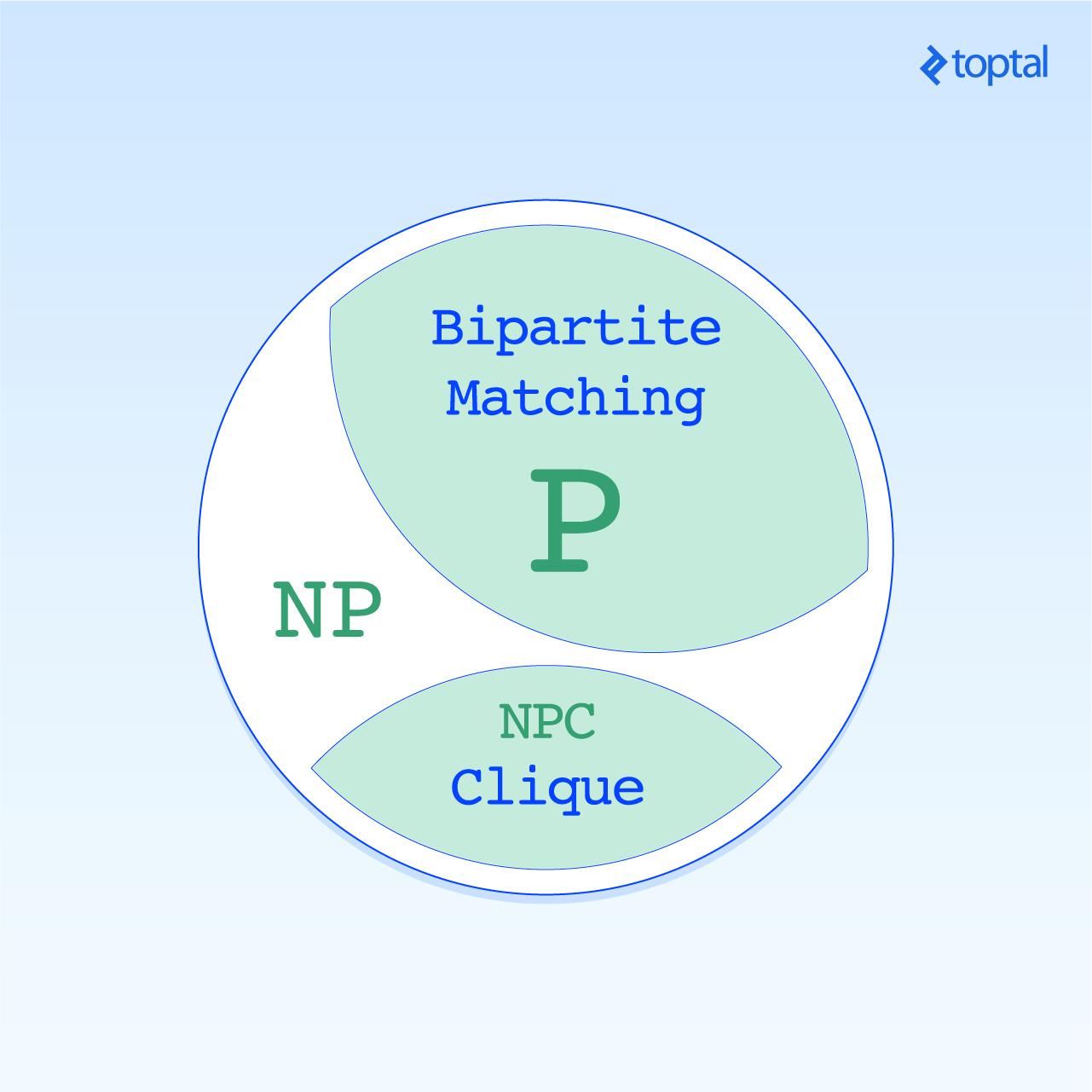Thus, we arrive at the problem of P vs. NP. Many computer scientists and mathematicians strongly believe that P and NP are not equal. If they were, the implications would be beyond profound. Much of today’s digital infrastructure relies on the fact that there are problems in NP that are not in P. If that were not the case, then cryptographic methods, for example, would collapse overnight, allowing a person possessing an efficient solution to an NPC problem to subvert even the tightest security protocols.

### Tractability Subtleties

To computer science novices, the difference between the matching and clique problems might not seem like a big deal. In fact, the difference between a problem in P and a problem in NP can be very subtle. Being able to tell the difference is important for anyone designing algorithms in the real world.

Consider the shortest path problem. Given two locations, the objective is to identify the shortest path between them. An iPhone computes this in a matter of milliseconds. This is a computationally tractable problem.

On the other hand, consider the traveling salesman problem, where the objective is to visit a subset of possible destinations ending at the origin while traveling the shortest possible distance. This problem is similar to the shortest path problem but is NP-complete; it also explains why supply chain logistics is a billion dollar industry.

We can actually be even subtler. Instead of asking for the shortest path (P), we can ask for the longest path without cycles. Turns out longest path problem is also NP-complete.

There are many more examples of this subtle distinction, including identification of vertex covers in bipartite vs. general graphs or satisfaction of boolean formulas with two vs. three literals per clause. The point is that it is not immediately obvious whether a problem is in P or NP, and this is why running time analysis is a critical skill. If the algorithm one must design is for a problem in P, then we know there is an efficient solution. If on the other hand the problem is in NP, then we have a strong case to argue against pursuing a solution, because the algorithm, generally, would simply take too long to solve the problem.

### Summary

In this examination of complexity, we defined the classes of problems P and NP. P informally represents problems that can be efficiently solved by a computer while NP represents those that are efficiently verifiable.

No one has been able to prove that P is not equal to NP. Whether these two classes of problems are equivalent is the known as the P vs. NP problem, and it is the most important open problem in theoretical computer science today if not in all of mathematics. In fact, in the year 2000, the Clay Math Institute named the P vs. NP problem as one of the seven most important open questions in mathematics and has offered a million-dollar bounty for a proof that determines the solution to this problem.

## Conclusion

In this article, we delved into the realms of computability and complexity, answering big questions such as, “What is a computer?” While the details can be overwhelming, there are a number of profound takeaways worth remembering:

• There are some things that simply cannot be computed, like the halting problem.

• There are some things that cannot be computed efficiently, like the problems in NPC.

More important than the details are the ways to think about computation and computational problems. In our professional lives and even in our day to day, we may come across problems never seen before, and we can use tried and true tools and techniques to determine the best course of action.

## Understanding the basics

### Are there computational problems without solutions?

Since the set of all valid programs is countable but the set of functions is not, then there must be some functions for which we simply cannot write programs.

### What is the Church-Turing Thesis?

The Church-Turing Thesis states that everything that is computable is computable by a Turing machine.

### What is the P versus NP problem?

It is the problem of confirming if every computational problem whose solution can be verified in polynomial time can also be solved in polynomial time.